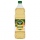Speedometer

The speedometer of a car reads 66 km/h. It's accurate to within 4.1%

What is the maximum possible error?

Result

x =  1.353 km/h

Solution:Leave us a comment of example and its solution (i.e. if it is still somewhat unclear...):Be the first to comment!Next similar examples:

1. Theorem proveWe want to prove the sentence: If the natural number n is divisible by six, then n is divisible by three. From what assumption we started?
2. Percentages52 is what percent of 93?
3. A perineumA perineum string is 10% shorter than its original string. The first string is 24, what is the 9th string or term?
4. Today in schoolThere are 9 girls and 11 boys in the class today. What is the probability that Suzan will go to the board today?
5. Three unknownsSolve the system of linear equations with three unknowns: A + B + C = 14 B - A - C = 4 2A - B + C = 0
6. Profit gainIf 5% more is gained by selling an article for Rs. 350 than by selling it for Rs. 340, the cost of the article is:
7. Percentage 1052 shorts and missed 13. Calculate percentage
8. Vinegar mixingWe mix 70g water and 50g 8% vinegar. How many percents of the solution of vinegar we produce?
9. BorrowingI borrow 25,000 to 6.9% p.a.. I pay 500 per month. How much will I pay and for how long?
10. LineIt is true that the lines that do not intersect are parallel?
11. Three workshopsThere are 2743 people working in three workshops. In the second workshop works 140 people more than in the first and in third works 4.2 times more than the second one. How many people work in each workshop?
12. BlocksThere are 9 interactive basic building blocks of an organization. How many two-blocks combinations are there?
13. TrigonometryIs true equality? ?
14. LossA bookstore purchased from a publisher the biography of a well-known politician for R15 per copy, but sales have been very poor. The manager has decided to mark the copies down to R12 each to make a quick sale. Calculate the loss on each book as a percenta
15. Sequence 2Write the first 5 members of an arithmetic sequence a11=-14, d=-1
16. LegsCancer has 5 pairs of legs. The insect has 6 legs. 60 animals have a total of 500 legs. How much more are cancers than insects?
17. SequenceWrite the first 7 members of an arithmetic sequence: a1=-3, d=6.